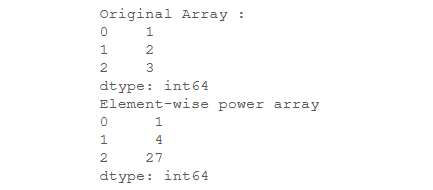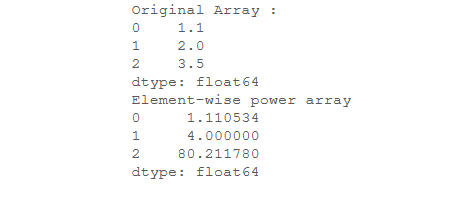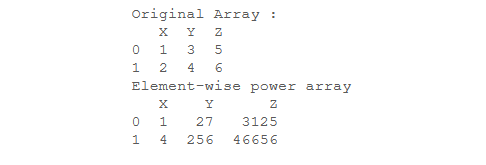Related Articles

# How to get the powers of an array values element-wise in Python-Pandas?

• Last Updated : 18 Aug, 2020

Let’s see how to get the powers of an array values element-wise. Dataframe/Series.pow() is used to calculate the power of elements either with itself or with other Series provided. This function is applicable for real numbers only, and doesn’t give results for complex numbers.
So let’s see the programs:

Example 1: The uni-dimensional arrays are mapped to a pandas series with either default numeric indices or custom indexes Then corresponding elements are raised to its own power.

## Python3

 `# import required modules``import` `numpy as np``import` `pandas as pd `` ` `# create an array``sample_array ``=` `np.array([``1``, ``2``, ``3``])  `` ` `# uni dimensional arrays can be``# mapped to pandas series``sr ``=` `pd.Series(sample_array) `` ` `print` `(``"Original Array :"``)``print` `(sr)`` ` `# calculating element-wise power ``power_array ``=` `sr.``pow``(sr)`` ` `print` `(``"Element-wise power array"``)``print` `(power_array)`

Output:Example 2: Powers can also be computed for floating-point decimal numbers.

## Python3

 `# module to work with arrays in python``import` `array`` ` `# module required to compute power``import` `pandas as pd`` ` `# creating a 1-dimensional floating ``# point array containing three elements``sample_array ``=` `array.array(``'d'``, ``                           ``[``1.1``, ``2.0``, ``3.5``])  `` ` `# uni dimensional arrays can ``# be mapped to pandas series``sr ``=` `pd.Series(sample_array) `` ` `print` `(``"Original Array :"``)``print` `(sr)`` ` `# computing power of each ``# element with itself ``power_array ``=` `sr.``pow``(sr)`` ` `print` `(``"Element-wise power array"``)``print` `(power_array)`

Output:Example 3: The Multi-dimensional arrays can be mapped to pandas data frames. The data frame then contains each cell comprising a numeric (integer or floating-point numbers) which can be raised to its own individual powers.

## Python3

 `# module to work with ``# arrays in python``import` `array`` ` `# module required to ``# compute power``import` `pandas as pd`` ` `# 2-d matrix containing ``# 2 rows and 3 columns``df ``=` `pd.DataFrame({``'X'``: [``1``,``2``],``                   ``'Y'``: [``3``,``4``],``                   ``'Z'``: [``5``,``6``]});`` ` `print` `(``"Original Array :"``)``print``(df)`` ` `# power function to calculate``# power of data frame elements``# with itself``power_array ``=` `df.``pow``(df)`` ` `print` `(``"Element-wise power array"``)``print` `(power_array)`

Output:Attention geek! Strengthen your foundations with the Python Programming Foundation Course and learn the basics.

To begin with, your interview preparations Enhance your Data Structures concepts with the Python DS Course. And to begin with your Machine Learning Journey, join the Machine Learning – Basic Level Course

My Personal Notes arrow_drop_up